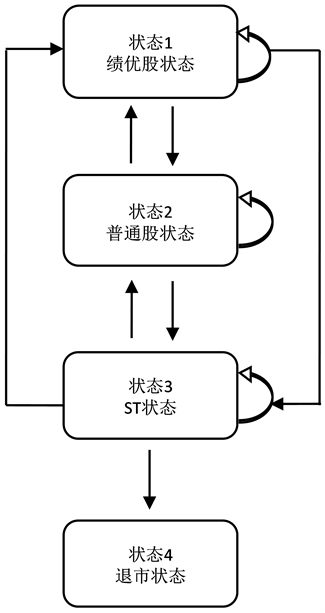基于多状态Markov模型的企业财务困境预测研究Research on Financial Distress Prediction Based on Multi-State Markov Model

• 全文下载: PDF(500KB)    PP.154-160   DOI: 10.12677/FIN.2018.84018
• 下载量: 286  浏览量: 387   国家科技经费支持

Based on the multi-state Markov model, the financial distress prediction problem of listed com-panies is studied. First, a multi-state Markov model is constructed. Then, based on the multi-state Markov model, we construct an exponential model with covariables of financial distress factors. Finally, the financial dilemma of listed companies is forecasted from the two aspects of transition probability and residence time. The results show that the multi-state Markov model can be applied to the prediction of financial distress and can reveal the evolution of financial distress.

1. 引言

2. 多状态Markov模型构建

3. 指数模型Figure 1. Multi-state Markov model transfer flow chart

(观察对象编号，状态，观察时刻，协变量1，协变量2……)

$Q=\left(\begin{array}{cccc}0& 0.5& 0.01& 0\\ 0.3& 0& 0.166& 0\\ 0.01& 0.01& 0& 0.04\\ 0& 0& 0& 0\end{array}\right)$ (1)

3.1. 多状态多因素Markov模型拟合结果

3.2. Markov指数模型

${q}_{ij}\left(Z\right)={q}_{ij0}\mathrm{exp}\left[{\sum }_{k=1}^{n}{\beta }_{ijk}{Z}_{k}\right]$ (2)Table 1. Multi-factor and multi-state Markov model fitting results

$\begin{array}{c}{q}_{12}\left(Z\right)={q}_{120}\mathrm{exp}\left[{\sum }_{k=1}^{n}{\beta }_{12k}{Z}_{k}\right]\\ =0.413\mathrm{exp}\left[0.502×总资产净利率-0.064×流动比率+1.383×营运资金比率\\ \text{\hspace{0.17em}}\text{\hspace{0.17em}}-2.202×营业收入现金比率-0.101×存货周转率\right]\end{array}$ .

$\begin{array}{c}{q}_{13}\left(Z\right)={q}_{130}\mathrm{exp}\left[{\sum }_{k=1}^{n}{\beta }_{13k}{Z}_{k}\right]\\ =0.005\mathrm{exp}\left[-0.365×总资产净利率-0.843×流动比率-0.600×营运资金比率\\ \text{\hspace{0.17em}}\text{\hspace{0.17em}}-0.160×营业收入现金比率-0.332×存货周转率\right]\end{array}$ .

$\begin{array}{c}{q}_{21}\left(Z\right)={q}_{210}\mathrm{exp}\left[{\sum }_{k=1}^{n}{\beta }_{21k}{Z}_{k}\right]\\ =0.081\mathrm{exp}\left[2.096×总资产净利率-0.649×流动比率+1.453×营运资金比率\\ \text{\hspace{0.17em}}\text{\hspace{0.17em}}+0.7441×营业收入现金比率+0.039×存货周转率\right]\end{array}$ .

$\begin{array}{c}{q}_{23}\left(Z\right)={q}_{230}\mathrm{exp}\left[{\sum }_{k=1}^{n}{\beta }_{23k}{Z}_{k}\right]\\ =0.017\mathrm{exp}\left[-2.007×总资产净利率+0.287×流动比率-0.989×营运资金比率\\ \text{\hspace{0.17em}}\text{\hspace{0.17em}}-0.144×营业收入现金比率-0.095×存货周转率\right]\end{array}$ .

$\begin{array}{c}{q}_{31}\left(Z\right)={q}_{310}\mathrm{exp}\left[{\sum }_{k=1}^{n}{\beta }_{31k}{Z}_{k}\right]\\ =0.001\mathrm{exp}\left[0.064×总资产净利率+0.252×流动比率+0.527×营运资金比率\\ \text{\hspace{0.17em}}\text{\hspace{0.17em}}-0.023×营业收入现金比率-0.005×存货周转率\right]\end{array}$ .

$\begin{array}{c}{q}_{32}\left(Z\right)={q}_{320}\mathrm{exp}\left[{\sum }_{k=1}^{n}{\beta }_{32k}{Z}_{k}\right]\\ =0.0007\mathrm{exp}\left[0.138×总资产净利率+0.372×流动比率+0.949×营运资金比率\\ \text{\hspace{0.17em}}\text{\hspace{0.17em}}+0.186×营业收入现金比率+0.0004×存货周转率\right]\end{array}$ .

$\begin{array}{c}{q}_{34}\left(Z\right)={q}_{340}\mathrm{exp}\left[{\sum }_{k=1}^{n}{\beta }_{34k}{Z}_{k}\right]\\ =-0.029\mathrm{exp}\left[0.397×总资产净利率-0.271×流动比率+1.135×营运资金比率\\ \text{\hspace{0.17em}}\text{\hspace{0.17em}}-0.111×营业收入现金比率-0.0004×存货周转率\right]\end{array}$ .

4. 运用多状态Markov模型进行预测

4.1. 转移概率Table 2. Matrix of transition probability (t = 1 year)Table 3. Matrix of transition probability (t = 2 year)Table 4. Matrix of transition probability (t = 5 year)Table 5. Matrix of transition probability (t = 10 year)

4.2. 总停留时间

${L}_{S}={\int }_{{t}_{1}}^{{t}_{2}}P{\left(t\right)}_{r,s}\text{d}t$ 。其中，LS表示在时刻t1和t2之间处于状态s的总停留时间，r为财务状态变化发展过

5. 研究结论

  Beaver, W.H. (1966) Financial Ratios as Predictors of Failure. Journal of Accounting Research, 4, 71-111. https://doi.org/10.2307/2490171  Altman, E.I. (1968) Financial Ratios Discriminant Analysis and the Prediction of Bankruptcy. Journal of Finance, No. 23, 589-609. https://doi.org/10.1111/j.1540-6261.1968.tb00843.x  Lau, A.H.-L. (1997) The Effects of Reducing Demand Uncertainty in a Manufacturer-Retailer Channel for Single-Period Products. Computers & OR (COR), 29, 1583-1602.  Morris, A. (1997) Genetical Gorithms Applications in the Analysis of Insolvency Risk. Journal of Banking and Finance, No. 22, 1401-1409.  Altman, E.I., Iwanicz-Drozdowska, M., Laitinen, E.K., et al. (2016) Financial Distress Prediction in an International Context: A Review and Empirical Analysis of Altman’s Z-Score Model. Journal of International Financial Man-agement & Accounting, 28, 131-171. https://doi.org/10.1111/jifm.12053  Liao, Q. and Mehdian, S. (2016) Measuring Financial Distress and Predicting Corporate Bankruptcy: An Index Approach. Review of Economic and Business Studies, 9, 33-51. https://doi.org/10.1515/rebs-2016-0024  杨青龙, 田晓春, 胡佩媛. 基于LASSO方法的企业财务困境预测[J]. 统计与决策, 2016(23): 170-173.  蔡玉兰, 钱崇秀, 董雪杰. 财务报表信息对企业财务困境的预测能力[J]. 预测, 2016(5): 48-54.  邹清明, 黄钟亿. 基于比例优势模型的上市公司财务困境预测研究[J]. 数理统计与管理, 2016(3): 536-549.  Serri, M., Caldarelli, G. and Cimini, G. (2016) How the Interbank Market Becomes Systemically Dangerous: An Agent-Based Network Model of Financial Distress Propagation. arXiv Preprint arXiv: 1611.04311.  李丽君, 刘杰, 孙世敏. 供应链视角下企业财务困境传递及控制问题研究[J]. 中国管理科学, 2016(6): 46-51.  Bhaskar, L.S., Krishnan, G.V. and Yu, W. (2017) Debt Covenant Vi-olations, Firm Financial Distress, and Auditor Actions. Contemporary Accounting Research, 34, 186-215. https://doi.org/10.1111/1911-3846.12241  Megginson, W.L., Meles, A., Sampagnaro, G., et al. (2016) Financial Distress Risk in Initial Public Offerings: How Much Do Venture Capitalists Matter? Journal of Corporate Finance, 26, 1-52.  孙宝文, 牛超群, 赵宣凯, 荆文君. 财务困境识别: 中国P2P平台的风险特征研究[J]. 中央财经大学学报, 2016(7): 32-43 + 55.  Ross, B. (2000) Financial Ratios and Different Failure Processes. Journal of Business Finance, No. 3, 18-24.